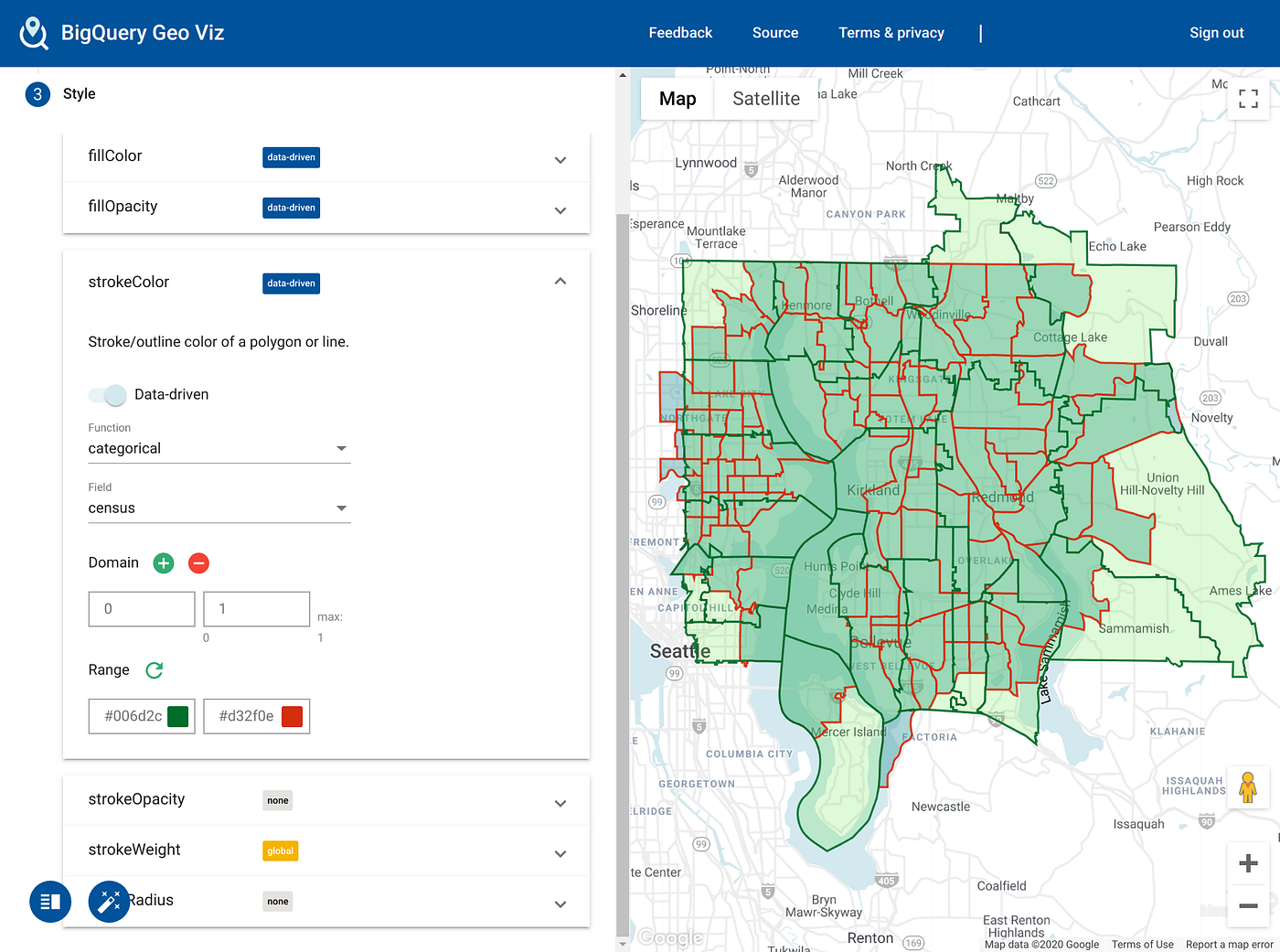# Mapping statistics between different spatial hierarchies

`select * from (  select     1 as census, tract_geom geom   from     `bigquery-public-data.geo_census_tracts.census_tracts_washington`  union all  select     0 as census, zip_code_geom geom   from     `bigquery-public-data.geo_us_boundaries.zip_codes`)where st_dwithin(geom, st_geogpoint(-122.191667, 47.685833), 5000)`Census tracts and zip code polygons
`CREATE OR REPLACE TABLE     demo.zip_tract_join ASWITH zip_tract_join AS (  SELECT     zips.zip_code,     zips.functional_status as zip_functional_status,    tracts.tract_ce,     tracts.geo_id as tract_geo_id,     tracts.functional_status as tract_functional_status,    ST_Area(ST_Intersection(tracts.tract_geom, zips.zip_code_geom))        / ST_Area(tracts.tract_geom) as tract_pct_in_zip_code  FROM      `bigquery-public-data.geo_census_tracts.us_census_tracts_national` tracts,    `bigquery-public-data.geo_us_boundaries.zip_codes` zips  WHERE     ST_Intersects(tracts.tract_geom, zips.zip_code_geom))SELECT * FROM zip_tract_join WHERE tract_pct_in_zip_code > 0;`
`SELECT geo_id, total_pop,        total_pop * income_per_capita as total_income FROM `bigquery-public-data.census_bureau_acs.censustract_2017_5yr``
`CREATE OR REPLACE TABLE demo.zip_pop_income ASWITH census_totals AS (  -- convert averages to additive totals  SELECT     geo_id, total_pop,     total_pop * income_per_capita AS total_income   FROM     `bigquery-public-data.census_bureau_acs.censustract_2017_5yr` ),joined AS (   -- join with precomputed census/zip pairs,  -- compute zip's share of tract  SELECT     zip_code,     total_pop * tract_pct_in_zip_code    AS zip_pop,    total_income * tract_pct_in_zip_code AS zip_income  FROM census_totals c  JOIN demo.zip_tract_join ztj  ON c.geo_id = ztj.tract_geo_id),sums AS (   -- aggregate all "pieces" of zip code  SELECT    zip_code,     SUM(zip_pop) AS zip_pop,    SUM(zip_income) AS zip_total_inc  FROM joined   GROUP BY zip_code)SELECT   zip_code, zip_pop,   -- convert to averages  zip_total_inc/zip_pop AS income_per_capitaFROM sums`
`with zipcodes_within_distance as (SELECT   zip_code, zip_code_geomFROM   `bigquery-public-data.geo_us_boundaries.zip_codes`WHERE  ST_DWithin(      ST_GeogPoint(-122.191667, 47.685833),      zip_code_geom,      1609 * 5))select   zip_code_geom, stats.*from   zipcodes_within_distance areajoin   demo.zip_pop_income statson area.zip_code = stats.zip_code`
`SELECT   income_per_capita, tract_geomFROM   `bigquery-public-data.geo_census_tracts.census_tracts_washington` tJOIN  `bigquery-public-data.census_bureau_acs.censustract_2017_5yr` sON  t.geo_id = s.geo_idWHERE  ST_DWithin(      ST_GeogPoint(-122.191667, 47.685833),      tract_geom,      1609 * 5)`

--

--## Michael Entin

Hi, I'm TL of BigQuery Geospatial project. Posting small recipes and various notes for BQ Geospatial users.